# Different ways to hide elements using CSS

• Last Updated : 02 May, 2022

While working on UI/UX we have to take care of every web element that appears on the webpage to make the user experience easier. While designing such pages we often need to hide or show some particular HTML elements on any event or on any particular interval. In this article, we could do that easily with some line of javascript code, but for this article, we will see how many ways are there to hide an HTML element using CSS only.

There are the following CSS properties use to hide an element.

1. Absolute position
2. Color
3. Clip-path
4. Display
5. filter
6. Measurements
7. Opacity
8. Transform
9. Visibility

We are going to see all the methods mentioned above for hiding elements along with the CSS codes.

1. Absolute position: When we use the Position: absolute property in CSS for an element then it simply means that the position of that element is fixed to its parent container, if there is no container available then the document body is used as its parent container. Now we can use Top, Bottom, Left, Right properties to change the position of that element. We will use one of these properties to hide the element from the document body.

Example: In this example, we will hide the text element by moving out it from the screen. We will set the position of the h1 tag to the absolute and on a button click, we will add a class in which we will use the left property and set the value to the -999px. By doing this the text will be moved out from the display. We can also use other Top, Bottom, and Right properties to hide the element from the document.

## HTML

 ```<``html` `lang``=``"en"``>` `<``head``>``    ``<``script` `src``=``"https://ajax.googleapis.com/ajax/libs/jquery/3.6.0/jquery.min.js"``>``    ``` `    ``<``style``>``        ``body {``            ``background-color: rgb(37, 37, 37);``        ``}` `        ``h1 {``            ``font-size: 3rem;``            ``color: green;``            ``position: absolute;``        ``}` `        ``p {``            ``color: white;``        ``}` `        ``h1.hide {``            ``left: -999px;``        ``}``    ````` `<``body``>``    ` `<``p``>``        ``This button will hide the text element``        ``by moving out the element from the screen.``    ```  `    ``<``button` `id``=``"btn"``>Hide` `    ``<``div` `class``=``"textbox"``>``        ``<``h1``>GeeksForGeeks``    ````    ` `    ``<``script``>``        ``\$("#btn").click(function () {` `            ``\$("h1").addClass("hide");``        ``});``    ````` ``

Output: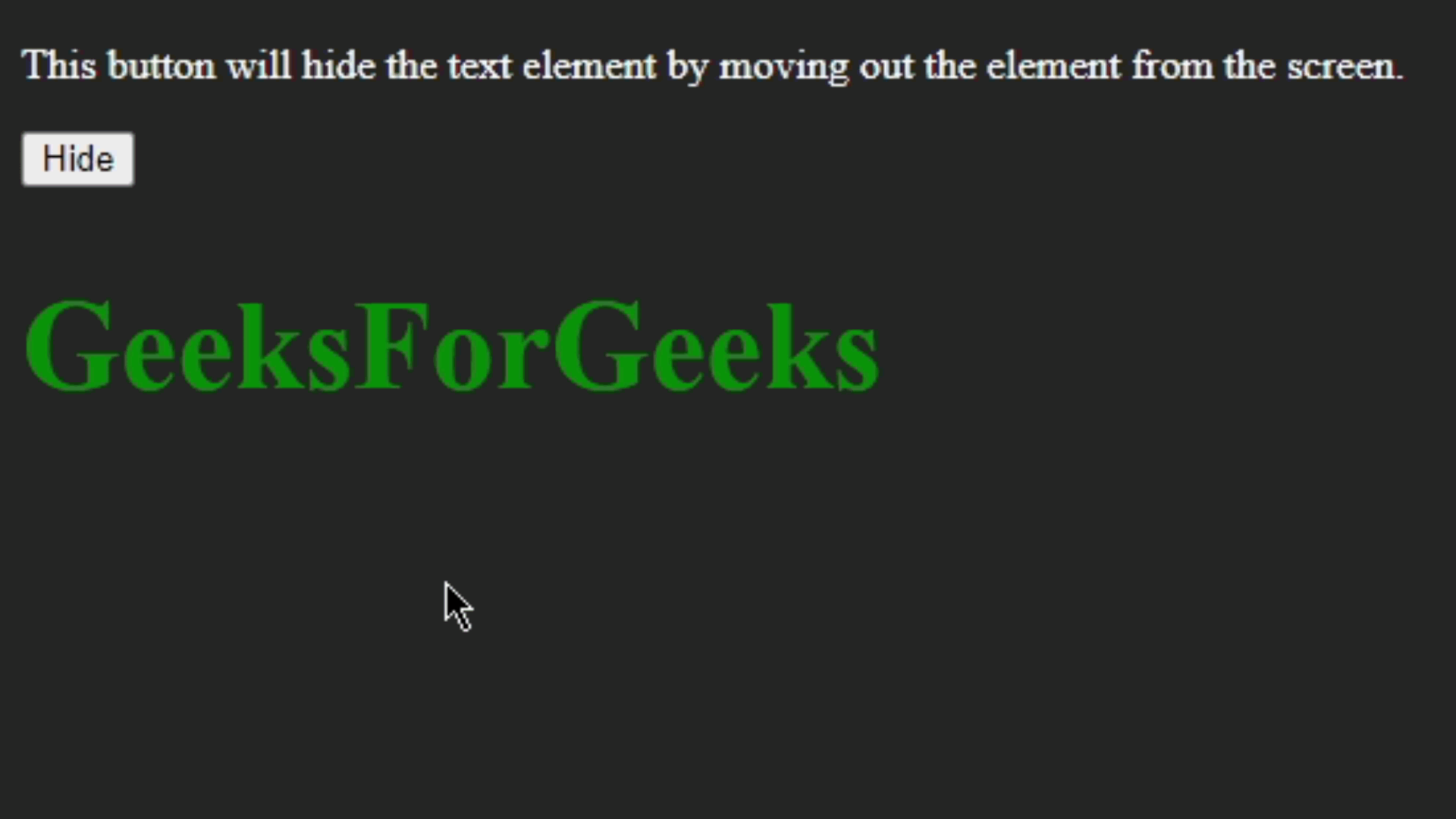2. Color property can also be used for making elements invisible by making them transparent, we will apply color with an alpha channel. We can set the alpha channel with the following method.

1. color: transparent
2. color: hsla(hue, saturation, lightness, alpha);
3. color: rgba(red, green, blue, alpha);
4. color: #RRGGBBAA
5. color: #RBGA

Note: We can also use these values other than color, for example to the background-color, border-color, background, etc.

Example: In this example, we will hide the element by making it transparent.

## HTML

 ```<``html` `lang``=``"en"``>` `<``head``>``    ``<``script` `src``=``"https://ajax.googleapis.com/ajax/libs/jquery/3.6.0/jquery.min.js"``>``    ``` `    ``<``style``>``        ``body {``            ``background-color: rgb(37, 37, 37);``        ``}` `        ``h1 {``            ``font-size: 3rem;``            ``color: rgb(255, 255, 255);``        ``}` `        ``p {``            ``color: white;``        ``}` `        ``h1.hide {``            ``color: transparent;``        ``}``    ````` `<``body``>` `    ` `<``p``>``        ``This button will set the text element``        ``color to the transparent and the text``        ``will be hidden.``    ```  `    ``<``button` `id``=``"btn"``>Hide` `    ``<``div` `class``=``"textbox"``>``        ``<``h1``>GeeksForGeeks``    ````    ` `    ``<``script``>``        ``\$("#btn").click(function () {` `            ``\$("h1").addClass("hide");``        ``});``    ````` ``

Output: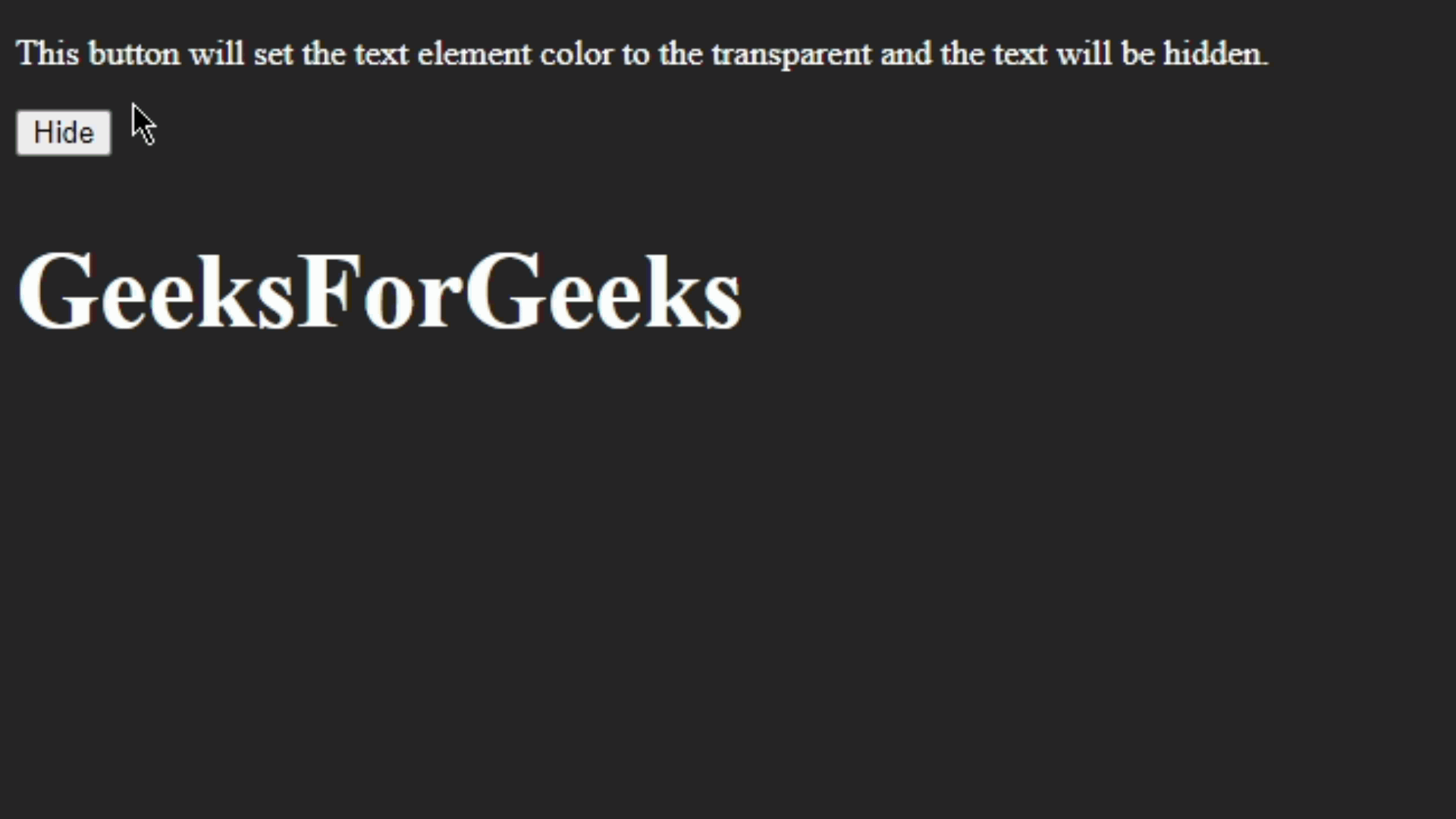3. Clip-path property: This property is used for creating a clipping reason according to the given shape, We will use this property to hide the element. Clip-path property has various values one of them is the circle(). when we use clip-path: circle(0)  then the particular element will be completely hidden.

Example: In this example, we are going to hide a div by using the clip-path property and we will use one of the value circle() and set it to the circle(0). By doing this it will completely clip out the div and the div will be hidden.

## HTML

 ```<``html` `lang``=``"en"``>` `<``head``>``    ``<``script` `src``=``"https://ajax.googleapis.com/ajax/libs/jquery/3.6.0/jquery.min.js"``>``    ``` `    ``<``style``>``        ``body {``            ``background-color: rgb(37, 37, 37);``        ``}` `        ``.rect {``            ``margin: 20px;``            ``height: 100px;``            ``width: 100px;``            ``background-color: #fff;``        ``}` `        ``p {``            ``color: white;``        ``}` `        ``.rect.hide {``            ``clip-path: circle(0);``        ``}``    ````` `<``body``>` `    ` `<``p``>``        ``This button will hide the text by``        ``clipping it in a circular shape to``        ``the zero value.``    ```  `    ``<``button` `id``=``"btn"``>Hide` `    ``<``div` `class``=``"textbox"``>``        ``<``div` `class``=``"rect"``>``    ````    ` `    ``<``script``>``        ``\$("#btn").click(function () {` `            ``\$(".rect").addClass("hide");``        ``});``    ````` ``

Output: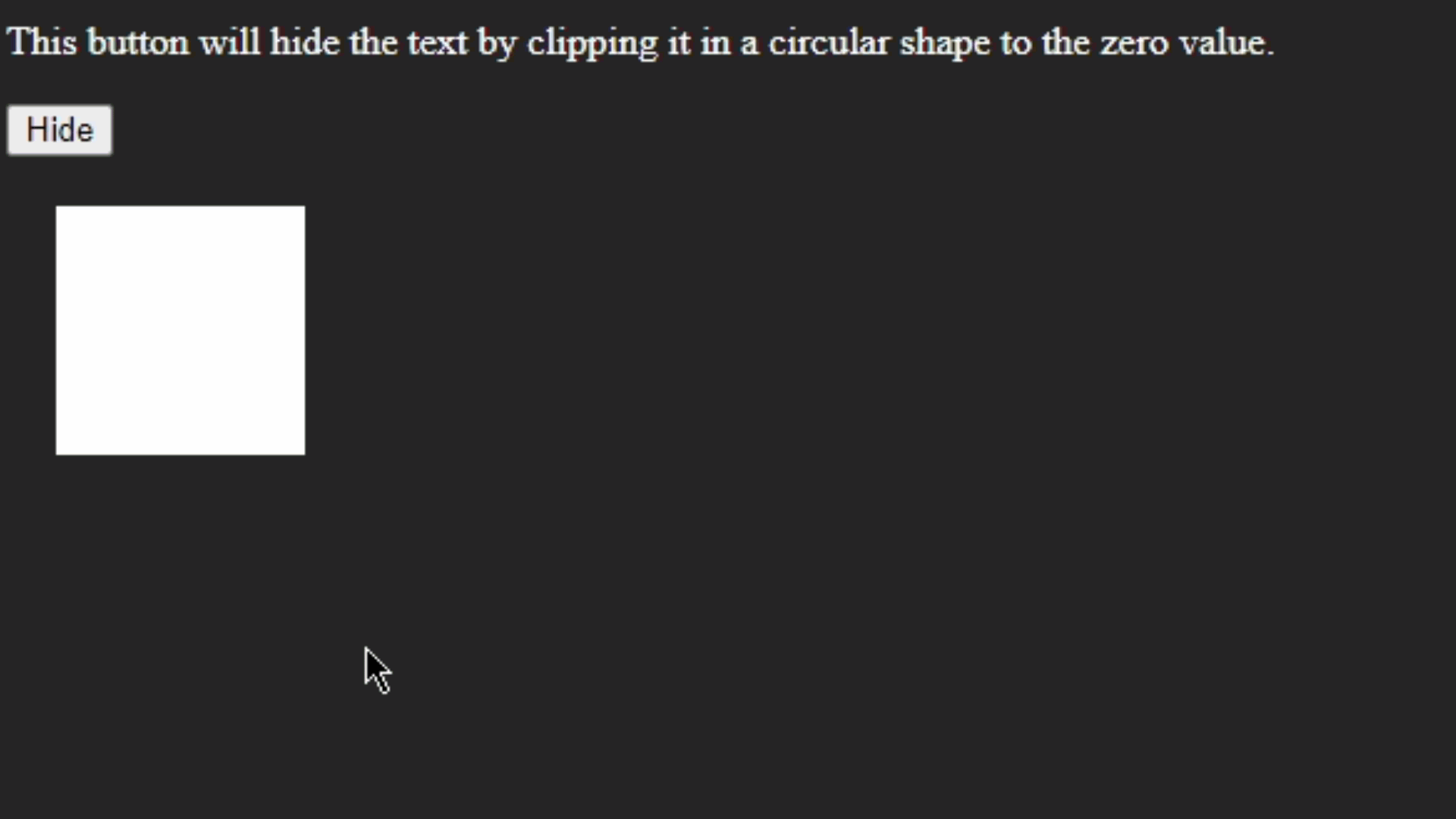4. Display property: The display property of CSS can also be used for hiding the element from DOM. We will use its one of the value which is known as Display: none, Probably this is the most used property for hiding HTML elements from the page.

Example: Hiding elements with display: none.

## HTML

 ```<``html` `lang``=``"en"``>` `<``head``>``    ``<``script` `src``=``"https://ajax.googleapis.com/ajax/libs/jquery/3.6.0/jquery.min.js"``>``    ``` `    ``<``style``>``        ``body {``            ``background-color: rgb(37, 37, 37);``        ``}` `        ``h1 {``            ``font-size: 3rem;``            ``color: rgb(24, 255, 63);``            ``border: 1px solid green;``            ``width: 17%;``        ``}` `        ``p {``            ``color: white;``        ``}` `        ``h1.hide {``            ``display: none;``        ``}``    ````` `<``body``>` `    ` `<``p``>``        ``This button will hide the text``        ``element by adding the class``        ``which specify "display:none"``        ``property``    ```  `    ``<``button` `id``=``"btn"``>Hide``    ` `    ``<``div` `class``=``"textbox"``>``        ``<``h1``>GeeksForGeeks``    ``` `    ``<``script``>``        ``\$("#btn").click(function () {``            ``\$("h1").addClass("hide");``        ``});``    ````` ``

Output: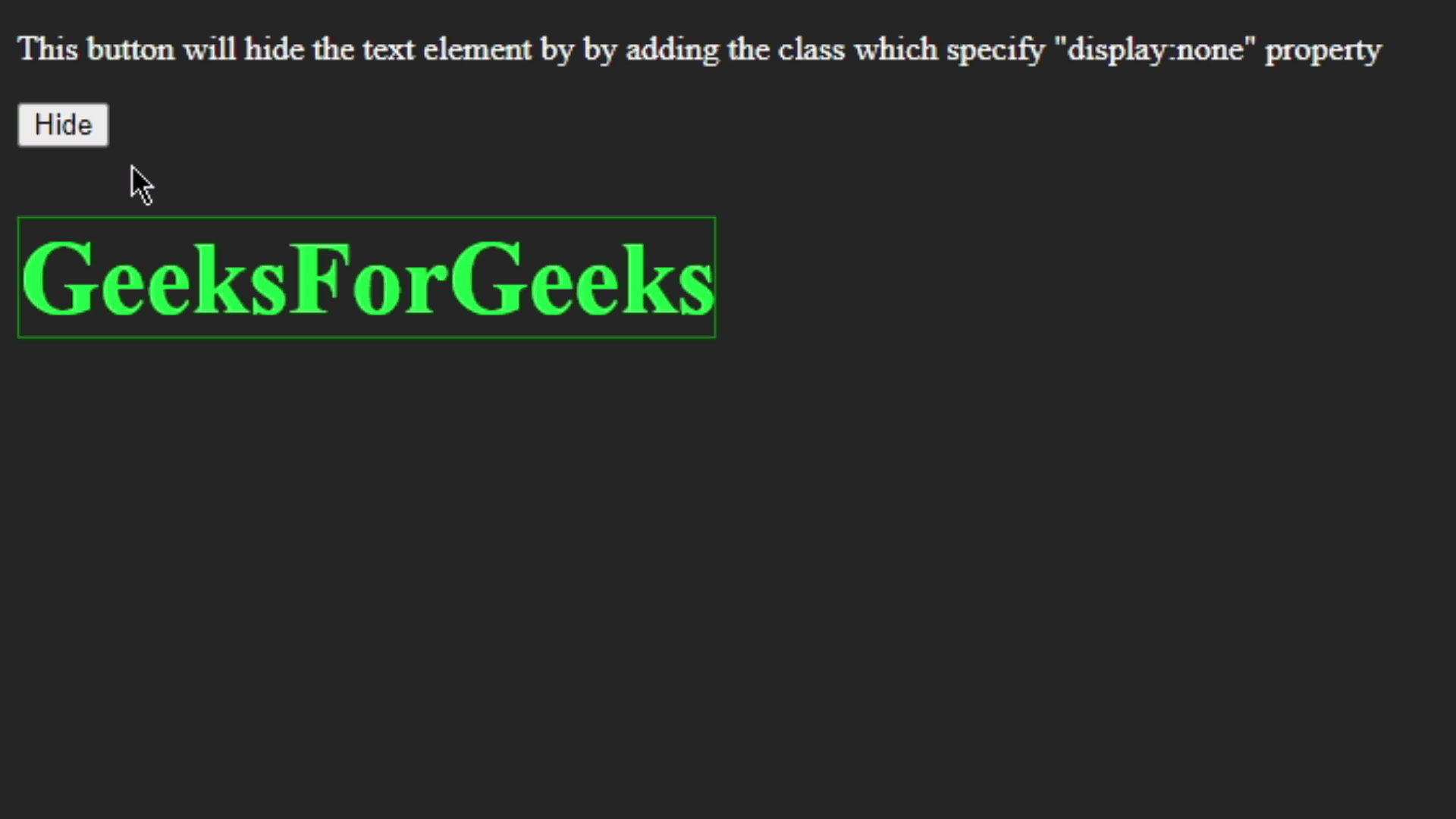5. Filter: The filter property in CSS is used for applying some graphical distortion to the HTML elements. Filter property has several values but for hiding the element we will use it’s one of the properties called opacity(). As the name suggests what will it do, We will use the lowest opacity value to make the element transparent and this way will be able to hide any particular element.

Example:  Hiding element with filter : opacity() property.

## HTML

 ```<``html` `lang``=``"en"``>` `<``head``>``    ``<``script` `src``=``"https://ajax.googleapis.com/ajax/libs/jquery/3.6.0/jquery.min.js"``>``    ``` `    ``<``style``>``        ``body {``            ``background-color: rgb(37, 37, 37);``        ``}` `        ``h1 {``            ``font-size: 3.5rem;``            ``color: rgb(24, 255, 63);``            ``border: 1px solid green;``            ``width: 20%;``        ``}` `        ``p {``            ``color: white;``        ``}` `        ``h1.hide {``            ``filter: opacity(0);``        ``}``    ````` `<``body``>` `    ` `<``p``>``        ``This button will hide the text by``        ``making its opacity to the zero.``    ```  `    ``<``button` `id``=``"btn"``>Hide``    ` `    ``<``div` `class``=``"textbox"``>``        ``<``h1``>GeeksForGeeks``    ``` `    ``<``script``>``        ``\$("#btn").click(function () {``            ``\$("h1").addClass("hide");``        ``});``    ````` ``

Output: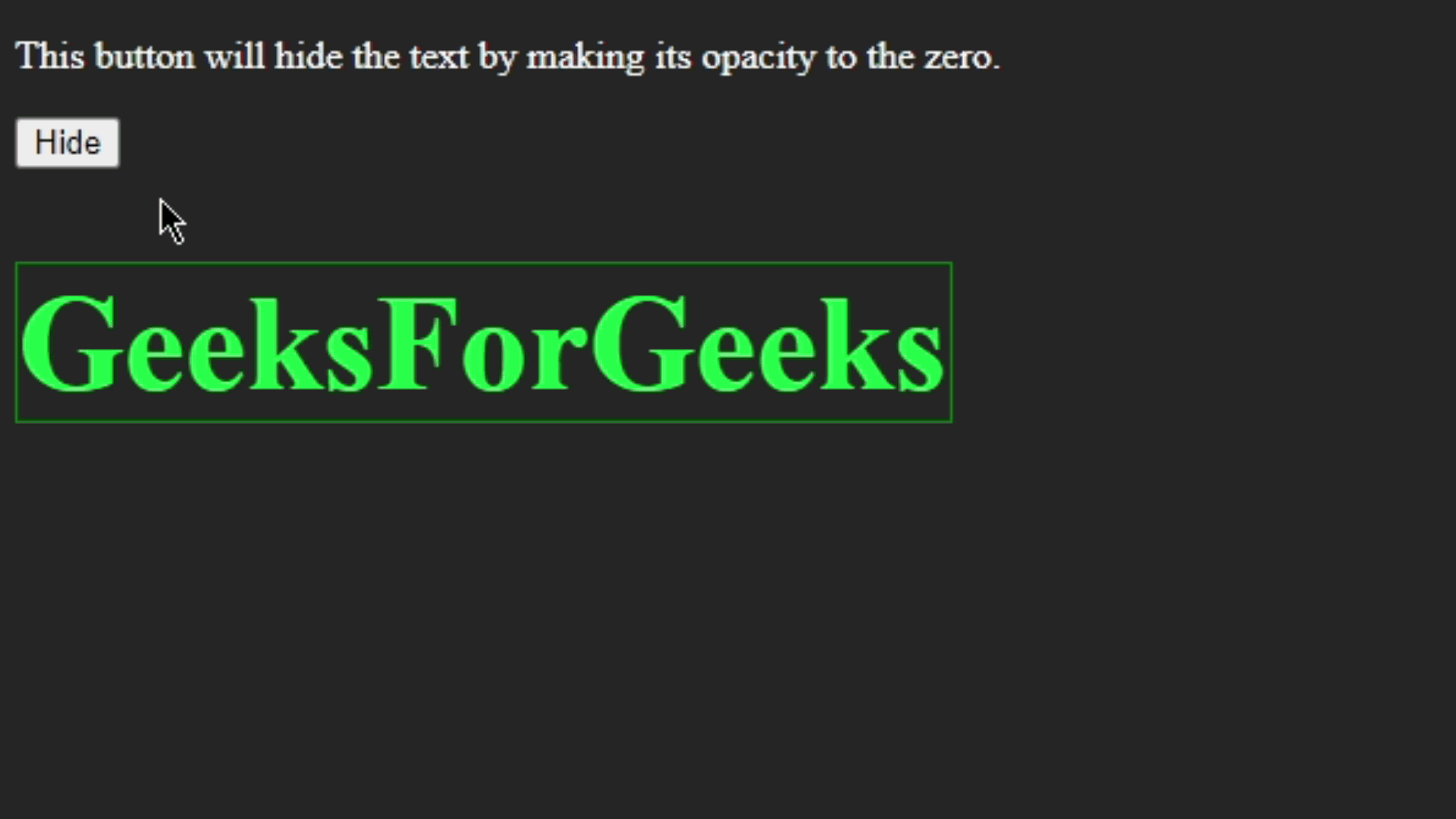6. Measurements: This could also be one of the simplest ways to hide any element. We will reduce the dimension of the element with the help of the properties like height, width, font size, etc. Note that when we use dimensions to reduce or completely hide the element don’t forget to use overflow: hidden property so that the complete content of the elements gets hidden.

Example: Hiding element by reducing its dimension.

## HTML

 ```<``html` `lang``=``"en"``>` `<``head``>``    ``<``script` `src``=``"https://ajax.googleapis.com/ajax/libs/jquery/3.6.0/jquery.min.js"``>``    ``` `    ``<``style``>``        ``body {``            ``background-color: rgb(37, 37, 37);``        ``}` `        ``h1 {``            ``font-size: 5rem;``            ``color: rgb(24, 255, 63);``            ``width: 20%;``        ``}` `        ``p {``            ``color: white;``        ``}` `        ``h1.hide {``            ``height: 0px;``            ``width: 0px;``            ``overflow: hidden;``        ``}``    ````` `<``body``>``    ` `<``p``>``        ``This button will hide the text by``        ``reducing its dimension to the zero``    ```  `    ``<``button` `id``=``"btn"``>Hide``    ` `    ``<``div` `class``=``"textbox"``>``        ``<``h1``>GeeksForGeeks``    ``` `    ``<``script``>``        ``\$("#btn").click(function () {``            ``\$("h1").addClass("hide");``        ``});``    ````` ``

Output: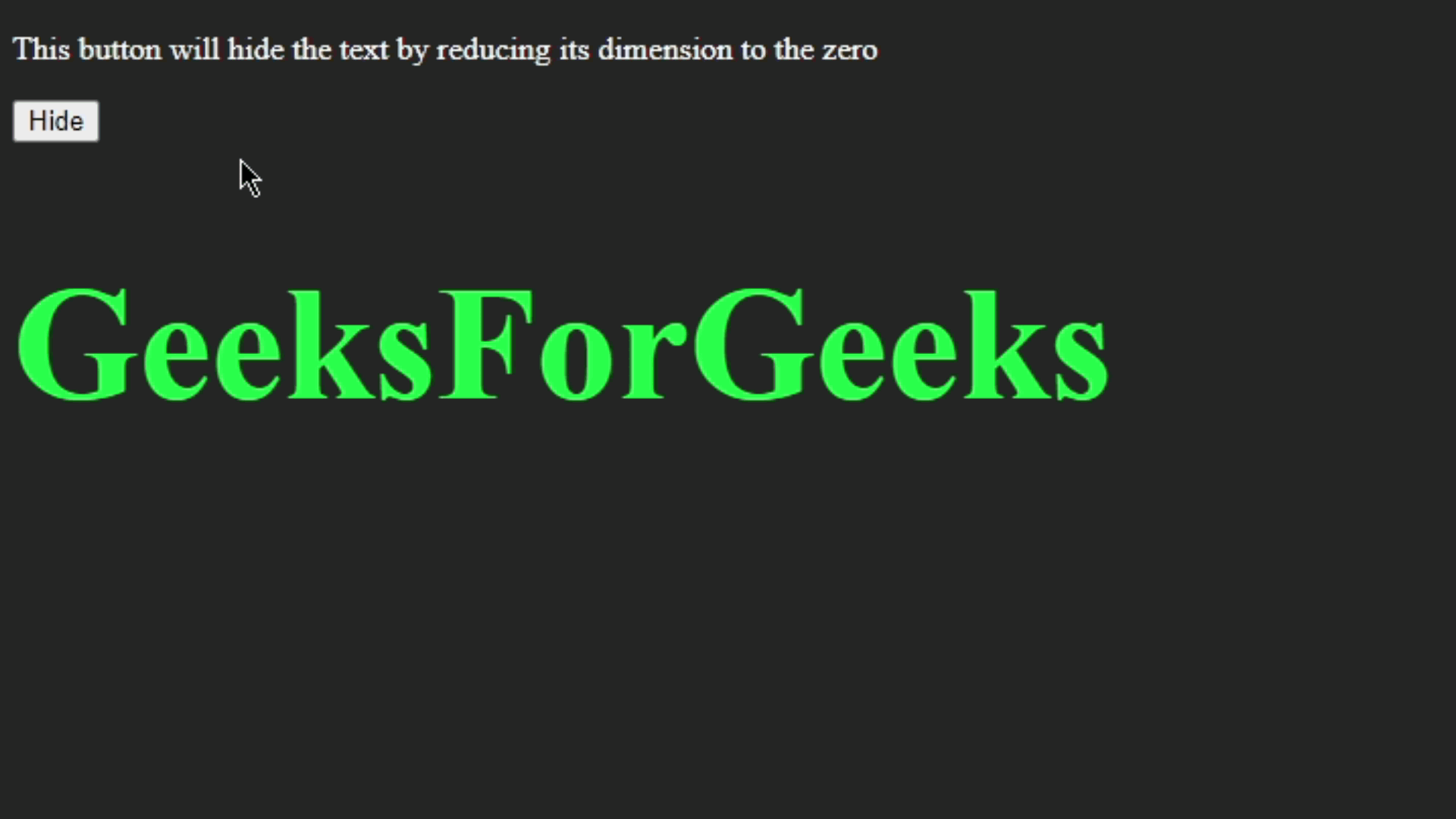7. Opacity: We can directly use the opacity property in CSS to apply a transparency effect to the element. We will use the opacity value from 0 to 1 or in the percentage from 0% to 100%. But in this case, we will use opacity(0) or opacity(0%) to make the element transparent or completely hide the element.

Example:  Hiding the element by setting opacity to 0.

## HTML

 ```<``html` `lang``=``"en"``>` `<``head``>``    ``<``script` `src``=``"https://ajax.googleapis.com/ajax/libs/jquery/3.6.0/jquery.min.js"``>``    ``` `    ``<``style``>``        ``body {``            ``background-color: rgb(37, 37, 37);``        ``}` `        ``h1 {``            ``font-size: 5rem;``            ``color: rgb(24, 255, 63);``            ``width: 20%;``        ``}` `        ``p {``            ``color: white;``        ``}` `        ``h1.hide {``            ``opacity: 0;``        ``}``    ````` `<``body``>``    ` `<``p``>``        ``This button will hide the text by``        ``making opacity of the text 0``    ```  `    ``<``button` `id``=``"btn"``>Hide``    ` `    ``<``div` `class``=``"textbox"``>``        ``<``h1``>GeeksForGeeks``    ``` `    ``<``script``>``        ``\$("#btn").click(function () {``            ``\$("h1").addClass("hide");``        ``});``    ````` ``

Output: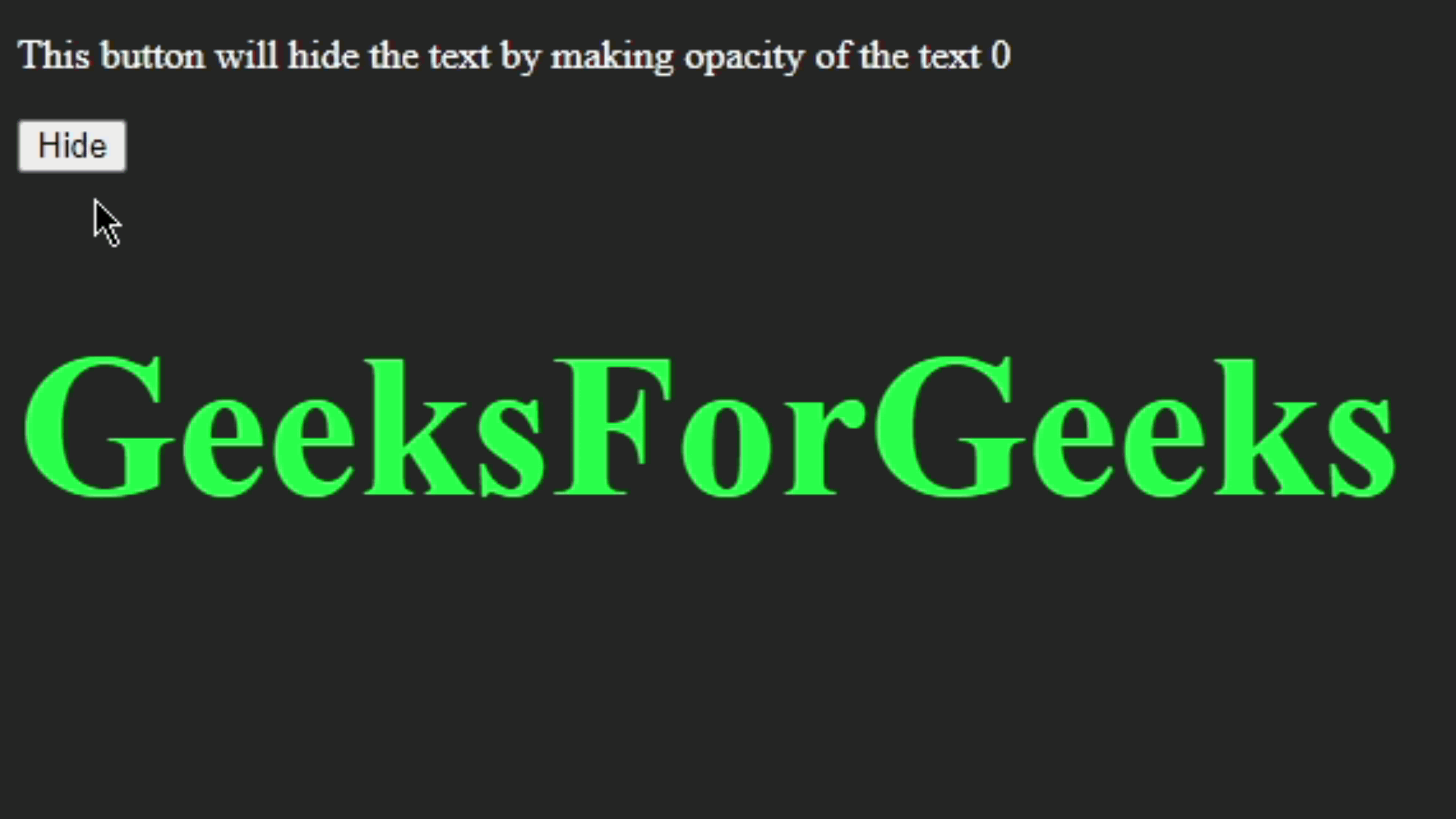8. Transform: The transform property of CSS can be used for scale, rotate, move the HTML element. For hiding the element from the document we will use scale() to hide the element. The scale() method will help to set the size of the element so we can completely hide the element by using the lowest value for scale().

Example: Hiding the element with Transform: scale().

## HTML

 ```<``html` `lang``=``"en"``>` `<``head``>``    ``<``script` `src``=``"https://ajax.googleapis.com/ajax/libs/jquery/3.6.0/jquery.min.js"``>``    ``` `    ``<``style``>``        ``body {``            ``background-color: rgb(37, 37, 37);``        ``}` `        ``.crcl {``            ``margin: 20px;``            ``height: 200px;``            ``width: 200px;``            ``border-radius: 100px;``            ``background-color: #fff;``        ``}` `        ``p {``            ``color: white;``        ``}` `        ``.crcl.hide {``            ``transform: scale(0);``        ``}``    ````` `<``body``>` `    ` `<``p``>``        ``This button will hide the shape``        ``by making their scale to the zero.``    ```  `    ``<``button` `id``=``"btn"``>Hide``    ` `    ``<``div` `class``=``"textbox"``>``        ``<``div` `class``=``"crcl"``>``    ``` `    ``<``script``>``        ``\$("#btn").click(function () {``            ``\$(".crcl").addClass("hide");``        ``});``    ````` ``

Output: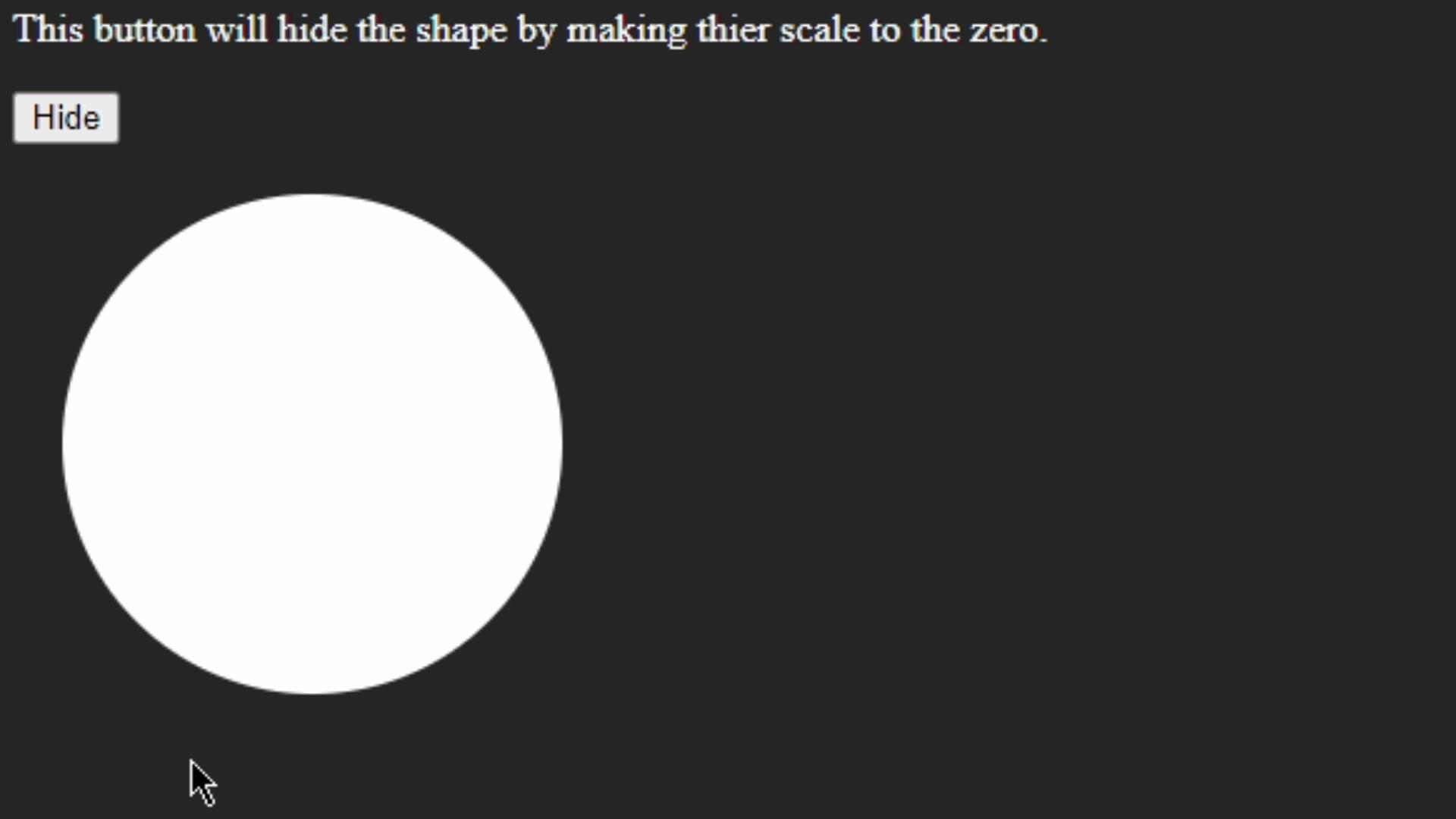9. Visibility: This property has only two values hidden and visible. For hiding elements from the page, we will simply use visibility: hidden. This is one of the simplest methods to hide the HTML element from DOM.

Example: Hiding element with visibility: hidden property

## HTML

 ```<``html` `lang``=``"en"``>` `<``head``>``    ``<``script` `src``=``"https://ajax.googleapis.com/ajax/libs/jquery/3.6.0/jquery.min.js"``>``    ``` `    ``<``style``>``        ``body {``            ``background-color: rgb(37, 37, 37);``        ``}` `        ``.crcl {``            ``margin: 20px;``            ``height: 200px;``            ``width: 200px;``            ``border-radius: 100px;``            ``background-color: rgb(3, 134, 10);``        ``}` `        ``p {``            ``color: white;``        ``}` `        ``.crcl.hide {``            ``visibility: hidden;``        ``}``    ````` `<``body``>``    ` `<``p``>``        ``This button will hide the shape by``        ``the property visibility: hidden``    ```  `    ``<``button` `id``=``"btn"``>Hide``    ` `    ``<``div` `class``=``"textbox"``>``        ``<``div` `class``=``"crcl"``>``    ``` `    ``<``script``>``        ``\$("#btn").click(function () {``            ``\$(".crcl").addClass("hide");``        ``});``    ````` ``

Output: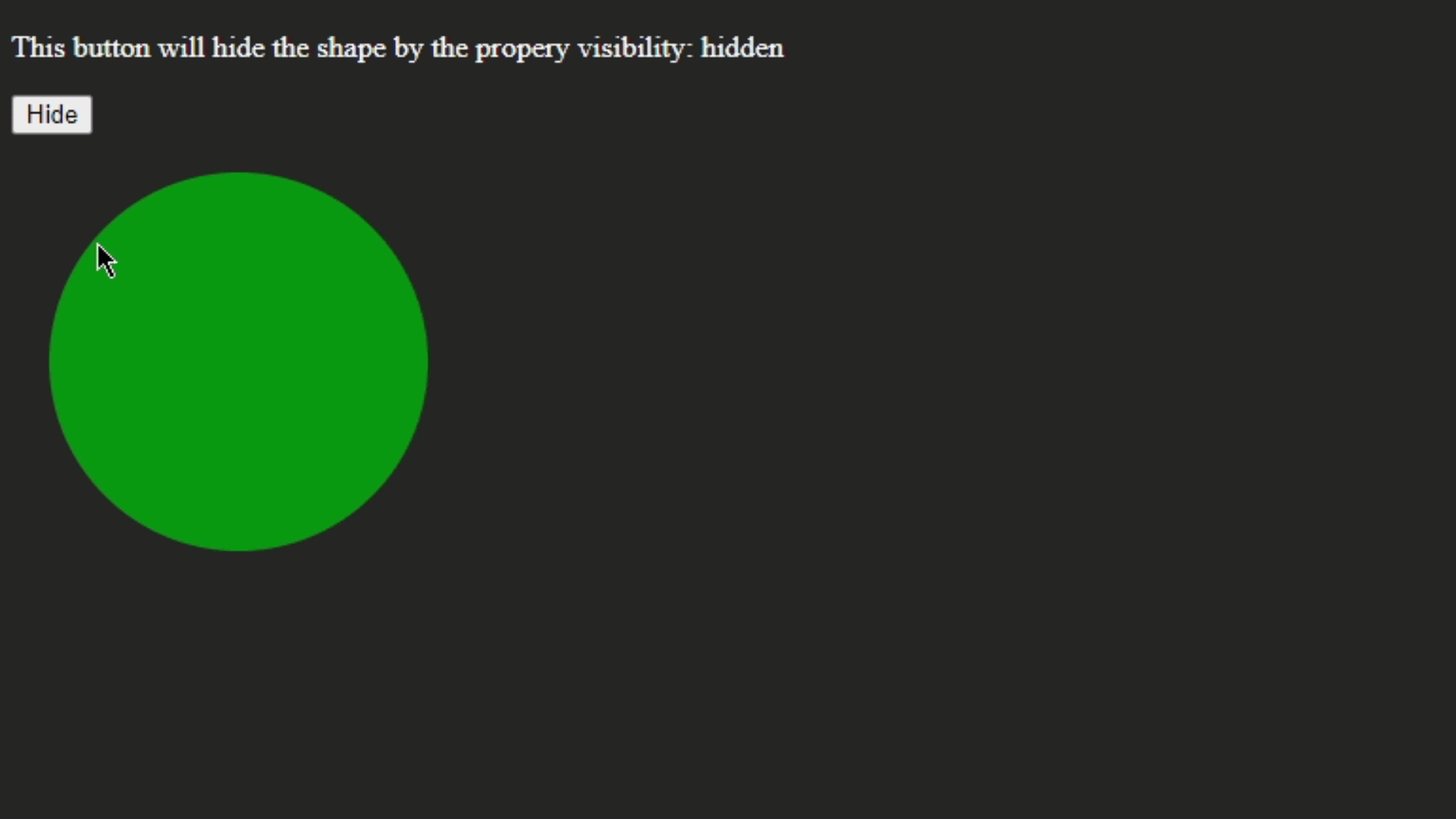My Personal Notes arrow_drop_up